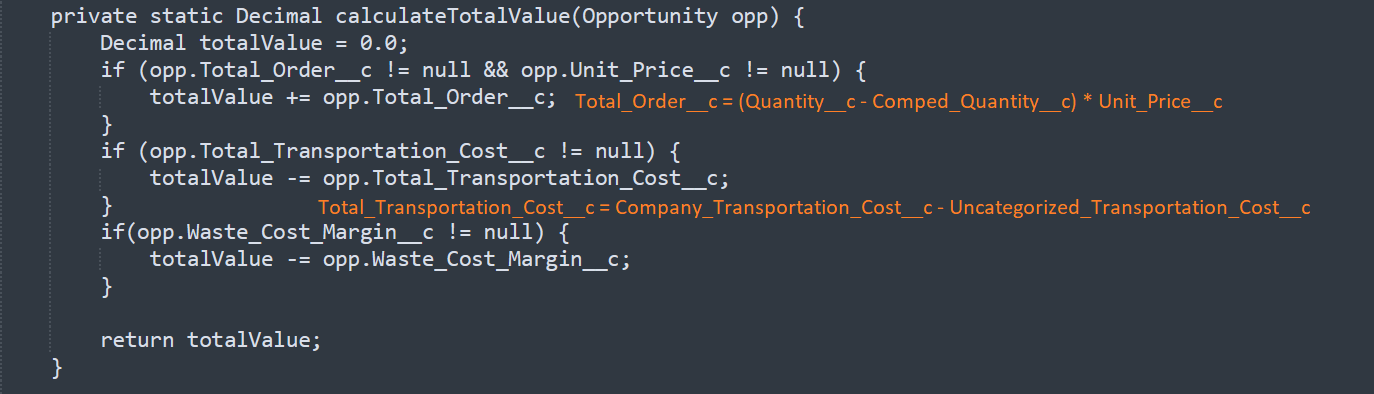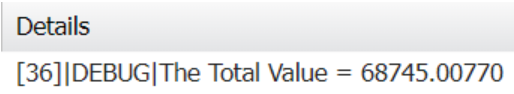Salesforce formula fields are a powerful and flexible tool to calculate values from related fields without needing the overhead of Flows or Apex code. However, when referencing them from before triggers, what makes them so efficient (calculating at runtime when accessed) may cause unexpected results if not handled carefully.

In this blog post, we’ll walk you through a case study that highlights how to get around the issue of formula fields in Apex triggers not evaluating correctly when values aren’t yet committed to the database.

Let’s get started!

## Case Study Scenario:

Let’s say that a retail business has a custom field on the Opportunity object called `Total_Value__c` that’s quite complex and requires Apex code to calculate. Their developers have written this calculation in the Opportunity before trigger, pulling together a number of fields from the Opportunity object and a related object, `Proposal__c`.

As good developers, they needed to support a couple formula fields that the company’s admins put together. So their calculation used both standard input fields and formula fields. They abstracted these calculations into a utility class, called `CalculateValues` which is called from the Opportunity trigger and looks something like the following:

``````/**
* This class's purpose is to automate Opportunity values based on proposal data. The
* Opportunity and Proposal are passed into two separate functions which (1) maps the
* data from the Proposal onto the Opportunity and (2) calculates the Total Value.
*/

public class CalculateValues {

public static void setProposalData(Opportunity opp, Proposal__c proposal) {
setDataFromProposal(opp, proposal);
opp.Total_Value__c = calculateTotalValue(opp);
}

private static void setDataFromProposal(Opportunity opp, Proposal__c proposal) {
opp.Unit_Price__c = proposal.Unit_Price__c;
opp.Quantity__c = proposal.Quantity__c;
opp.Comped_Quantity__c = proposal.Comped__c;
opp.Company_Transportation_Cost__c = proposal.Company_Transportation_Cost__c;
opp.Uncategorized_Transportation_Costs__c = proposal.Uncategorized_Transportation_Cost__c;
opp.Waste_Cost_Margin__c = proposal.Waste_Cost_Margin__c;
}

private static Decimal calculateTotalValue(Opportunity opp) {
Decimal totalValue = 0.0;
if (opp.Total_Order__c != null && opp.Unit_Price__c != null) {
totalValue += opp.Total_Order__c;
}
if (opp.Total_Transportation_Costs__c != null) {
totalValue -= opp.Total_Transportation_Costs__c;
}
if (opp.Waste_Cost_Margin__c != null) {
totalValue -= (totalValue * opp.Waste_Cost_Margin__c);
}
}
}
``````

But here’s the kicker: `Total_Order__c` and `Total_Transportation_Cost__c` are both formula fields. Here’s a screenshot of the formula next to where they are referenced in the code:Here’s an example test class for this calculation that asserts what you might expect the results for the `Total_Value__c` fields to be based on the above code:

``````@isTest
private class CalculateValues_Test {

private static Account acc;
private static Opportunity opp;

private static void init() {
acc = new Account(Name = 'TestAccount');
insert acc;
opp = new Opportunity(Name = 'Test Opp', AccountId = acc.Id, StageName = 'Qualification', CloseDate = Date.today().addMonths(2));
insert opp;
}

@isTest
private static void calculateValuesTest() {

init();

Test.startTest();

Proposal__c prop1 = new Proposal__c(Opportunity__c = opp.Id);
prop1.Unit_Price__c = 1.50;
prop1.Quantity__c = 50000;
prop1.Comped__c = 1400;
prop1.Company_Transportation_Cost__c = 4589.76;
prop1.Uncategorized_Transportation_Cost__c = 780.22;
prop1.Waste_Cost_Margin__c = 0.005;
insert prop1;

opp.Accepted_Proposal__c = prop1.Id;
update opp;
opp = getOpp(opp.Id);
System.assertEquals(1.50, opp.Unit_Price__c);
System.assertEquals(50000, opp.Quantity__c);
System.assertEquals(1400, opp.Comped_Quantity__c);
System.assertEquals(4589.76, opp.Company_Transportation_Cost__c);
System.assertEquals(780.22, opp.Uncategorized_Transportation_Costs__c);
System.assertEquals(0.005, opp.Waste_Cost_Margin__c);
System.assertEquals(((50000 - 1400) * 1.50) - (4589.76 - 780.22) - ((((50000 - 1400) * 1.50) - (4589.76 - 780.22))*0.005), opp.Total_Value__c);

Test.stopTest();
}

private static Opportunity getOpp(Id oppId) {
return [SELECT Id, Total_Order__c, Total_Value__c, Total_Transportation_Costs__c, Quantity__c,
Comped_Quantity__c, Company_Transportation_Cost__c, Uncategorized_Transportation_Costs__c,
Waste_Cost_Margin__c, Unit_Price__c
FROM Opportunity WHERE Id = :oppId];
}
}
``````

But when the test class runs….…the assertion fails. But, why?

The formula fields for `Total_Order__c` and `Total_Transportation_Cost__c` ran when the trigger was first called. Uploading the proposal, however, affects the resulting value of these fields.

Formula fields rely on data that has already been committed to the database, and because the changes made by the uploaded proposal are not yet committed to the database, the data that Salesforce loads for `Total_Order__c` and `Total_Transportation_Cost__c` is stale.

After update, if you were to run the calculation again with no additional changes made to the underlying fields, the formula fields would reflect correct values and the Total Value Calculation would be as expected. This is because the after trigger occurs after database commit and reloads any formula field values.

While it’s great to know that the formula does work, it doesn’t help us to make sure that our calculation is evaluating as expected in the before-context.

## The Solution:

The good news is there are some strategies you can use to get around this limitation of the formula fields. Here’s an example of a manual calculation strategy to do just that:

``````/**
* This class's purpose is to automate Opportunity values based on proposal data. The
* Opportunity and Proposal are passed into two separate functions which (1) maps the
* data from the Proposal onto the Opportunity and (2) calculates the Total Value.
*/

public class CalculateValues {

public static void setProposalData(Opportunity opp, Proposal__c proposal) {
setDataFromProposal(opp, proposal);
opp.Total_Value__c = calculateTotalValue(opp);
}

private static void setDataFromProposal(Opportunity opp, Proposal__c proposal) {
opp.Unit_Price__c = proposal.Unit_Price__c;
opp.Quantity__c = proposal.Quantity__c;
opp.Comped_Quantity__c = proposal.Comped__c;
opp.Company_Transportation_Cost__c = proposal.Company_Transportation_Cost__c;
opp.Uncategorized_Transportation_Costs__c = proposal.Uncategorized_Transportation_Cost__c;
opp.Waste_Cost_Margin__c = proposal.Waste_Cost_Margin__c;
}

private static Decimal calculateTotalValue(Opportunity opp) {
Decimal totalValue = 0.0;
if (opp.Total_Order__c != null && opp.Unit_Price__c != null) {
totalValue += calcTotalOrder(opp);
}
if (opp.Total_Transportation_Costs__c != null) {
totalValue -= calcTotalTransportationCost(opp);
}
if (opp.Waste_Cost_Margin__c != null) {
totalValue -= totalValue * opp.Waste_Cost_Margin__c;
}
}

private static Decimal calcTotalOrder(Opportunity opp) {
Decimal totalOrder = 0.0;
if (opp.Comped_Quantity__c != null) {
totalOrder += (opp.Quantity__c - opp.Comped_Quantity__c) * opp.Unit_Price__c;
} else {
totalOrder += opp.Quantity__c * opp.Unit_Price__c;
}
}

private static Decimal calcTotalTransportationCost(Opportunity opp) {
Decimal totalTransportationCost = 0.0;
if (opp.Uncategorized_Transportation_Costs__c != null) {
totalTransportationCost += opp.Company_Transportation_Cost__c - opp.Uncategorized_Transportation_Costs__c;
} else {
totalTransportationCost += opp.Company_Transportation_Cost__c;
}
}
}
``````

While the formula fields rely on data that has already been committed to the database, the new values mapped by the `setDataFromProposal` method are available to use in the `calculateTotalValue` calculation.

Instead of using the formula fields, the manual calculation breaks out the fields inside of the formula field and performs the calculation from each formula inside of two helper functions.

Now if we take that same test class from earlier and run it with our manually calculated Total Value calculation……the test now passes and the `Total_Value__c` field shows an accurate value.

## Limitations

The main limitation to this solution is that it forces you to replicate the formula field in Apex. You would have to be aware of any changes to this field and synchronize them with changes to the underlying code.